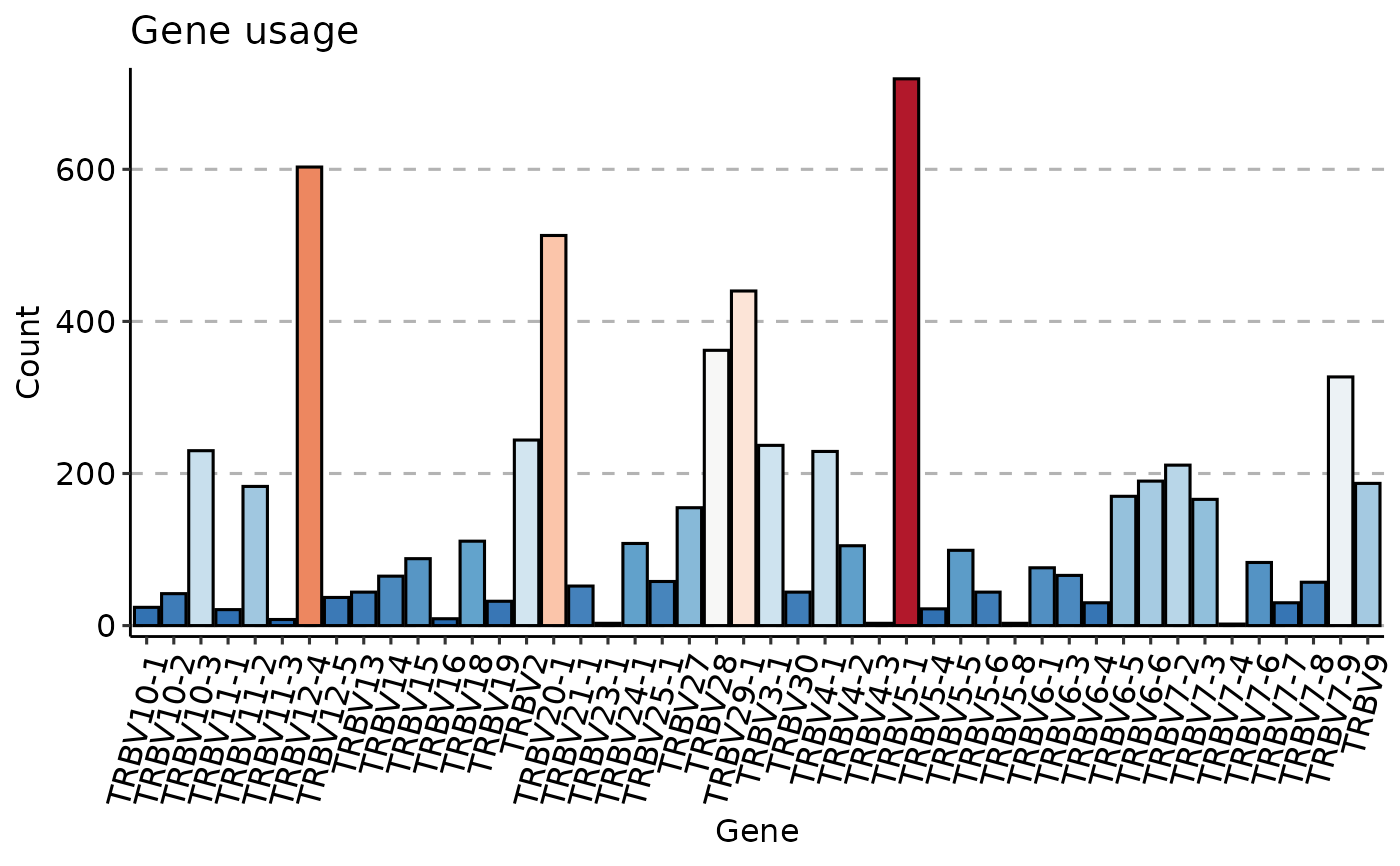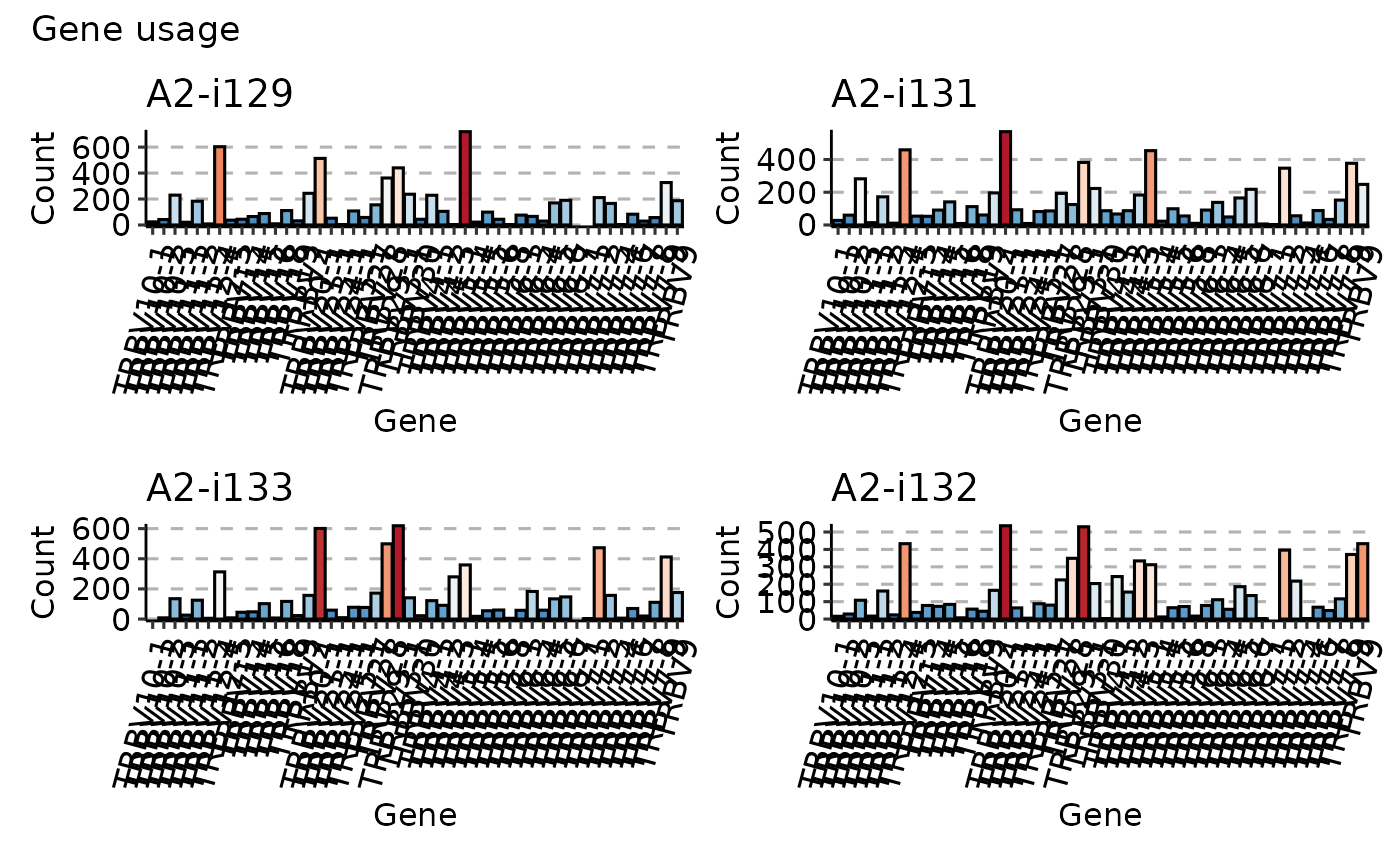Visualisation of distributions using ggplot2-based histograms.

vis_hist(
.data,
.by = NA,
.meta = NA,
.title = "Gene usage",
.ncol = NA,
.points = TRUE,
.test = TRUE,
.coord.flip = FALSE,
.grid = FALSE,
.labs = c("Gene", NA),
.melt = TRUE,
.legend = NA,
...
)

## Arguments

.data

Input matrix or data frame.

.by

Pass NA if you want to plot samples without grouping.

You can pass a character vector with one or several column names from ".meta" to group your data before plotting. In this case you should provide ".meta".

You can pass a character vector that exactly matches the number of samples in your data, each value should correspond to a sample's property. It will be used to group data based on the values provided. Note that in this case you should pass NA to ".meta".

.meta

A metadata object. An R dataframe with sample names and their properties, such as age, serostatus or hla.

.title

The text for the title of the plot.

.ncol

A number of columns to display. Provide NA (by default) if you want the function to automatically detect the optimal number of columns.

.points

A logical value defining whether points will be visualised or not.

.test

A logical vector whether statistical tests should be applied. See "Details" for more information.

.coord.flip

If TRUE then swap x- and y-axes.

.grid

If TRUE then plot separate visualisations for each sample.

.labs

A character vector of length two with names for x-axis and y-axis, respectively.

.melt

If TRUE then apply melt to the ".data" before plotting. In this case ".data" is supposed to be a data frame with the first character column reserved for names of genes and other numeric columns reserved to counts or frequencies of genes. Each numeric column should be associated with a specific repertoire sample.

.legend

If TRUE then plots the legend. If FALSE removes the legend from the plot. If NA automatically detects the best way to display legend.

Addditional ggplot2 layers, that added to each plot in the output plot or grid of plots.

...

Is not used here.

## Value

A ggplot2 object.

## Details

If data is grouped, then statistical tests for comparing means of groups will be performed, unless .test = FALSE is supplied. In case there are only two groups, the Wilcoxon rank sum test (https://en.wikipedia.org/wiki/Wilcoxon_signed-rank_test) is performed (R function wilcox.test with an argument exact = FALSE) for testing if there is a difference in mean rank values between two groups. In case there more than two groups, the Kruskal-Wallis test (https://en.wikipedia.org/wiki/Kruskal A significant Kruskal-Wallis test indicates that at least one sample stochastically dominates one other sample. Adjusted for multiple comparisons P-values are plotted on the top of groups. P-value adjusting is done using the Holm method (https://en.wikipedia.org/wiki/Holm You can execute the command ?p.adjust in the R console to see more.

## Examples

data(immdata)
imm_gu <- geneUsage(immdata$data[]) vis(imm_gu, .plot = "hist", .add.layer = theme(axis.text.x = element_text(angle = 75, vjust = 1)) ) #> Using Names as id variablesimm_gu <- geneUsage(immdata$data[1:4])
vis(imm_gu,
.plot = "hist", .grid = TRUE, .add.layer =
theme(axis.text.x = element_text(angle = 75, vjust = 1))
)
#> Using Names as id variables
#> Warning: Removed 2 rows containing missing values (position_stack()).
#> Warning: Removed 2 rows containing missing values (position_stack()).
#> Warning: Removed 1 rows containing missing values (position_stack()).